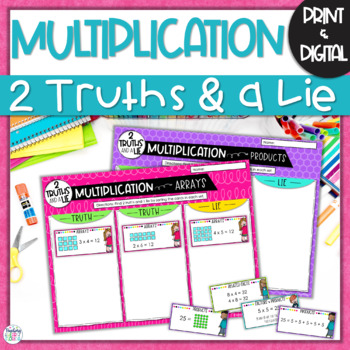# Multiplication Activity | Multiplication Math Center | 2 Truths & a Lie

Rated 4.71 out of 5, based on 7 reviews
7 Ratings;
2nd - 4th
Subjects
Resource Type
Standards
Formats Included
• Zip
•Google Apps™
Pages
30 pages
Report this resource to TPTThe Teacher-Author indicated this resource includes assets from Google Workspace (e.g. docs, slides, etc.).

#### What educators are saying

I absolutely love this game. It’s very engaging. East to prep and I love that it has answer sheets so students are able to check their work
##### Also included in
1. Engage your 3rd-grade students with this fun and rigorous math activity bundle throughout your multiplication unit! It's perfect for whole-class activities, small group interventions, math stations, fast finisher activities, homework, and review. You will save so much time planning your multiplicati
Price \$16.40Original Price \$20.50Save \$4.10

### Description

Your students will love this fun and engaging hands-on multiplication activity that helps them practice multiplication strategies such as arrays, related facts, and equal groups through error analysis! This QUICK PRINT AND GO or DIGITAL activity also gives students important practice with math vocabulary.

How to Use this Multiplication Activity:

Students are given 3 multiplication representations (example: arrays, equal groups, equations) and must determine which 2 are true and which one is a lie.

⭐ There are 5 different activities in this resource that help students practice with arrays, finding the product, equal groups, related facts, and using math vocabulary (factors and products).

⭐ This 2 Truths and a Lie Multiplication Error Analysis activity has so much flexibility in a printer friendly black and white version or color printables, and a digital version in Google™ Slides. Use it whichever way is best for YOU and your STUDENTS:

★ Cut and paste math centers activity

★ Print and laminate the pieces and use multiple times

★ Assign the digital version in a computer center.

→→→ PLEASE SEE PREVIEW FOR A CLOSER LOOK!

───────────────────────────

What's Included in this Resource:

Two Truths and a Lie Organizer Sheet, Multiplication Representation Cards, and Answer Key for:

• Arrays
• Products
• Equal Groups
• Factors & Products
• Related Facts

───────────────────────────

2 Truths and a Lie Multiplication Activities can easily be used in these settings:

• Whole Group Instruction
• Small Group Instruction
• Centers
• Formative Assessment
• Partner Work
• Bell Work

───────────────────────────
Common Core Math Standards Addressed in this Resource:
3.OA.A.1 - Interpret products of whole numbers, e.g., interpret 5 × 7 as the total number of objects in 5 groups of 7 objects each.
3.OA.B.5 - Apply properties of operations as strategies to multiply and divide.

───────────────────────────

If you and your students love this fun and engaging error analysis activity for multiplication, you will love these too!

Need more engaging and rigorous multiplication resources?

"Don't forget to leave feedback -you will receive TPT credits that you can use toward future purchases!

Thank you for visiting Teaching in the Heart of Florida!

Total Pages
30 pages
Included
Teaching Duration
Other
Report this resource to TPT
Reported resources will be reviewed by our team. Report this resource to let us know if this resource violates TPT’s content guidelines.

### Standards

to see state-specific standards (only available in the US).
Interpret products of whole numbers, e.g., interpret 5 × 7 as the total number of objects in 5 groups of 7 objects each. For example, describe a context in which a total number of objects can be expressed as 5 × 7.
Apply properties of operations as strategies to multiply and divide. Examples: If 6 × 4 = 24 is known, then 4 × 6 = 24 is also known. (Commutative property of multiplication.) 3 × 5 × 2 can be found by 3 × 5 = 15, then 15 × 2 = 30, or by 5 × 2 = 10, then 3 × 10 = 30. (Associative property of multiplication.) Knowing that 8 × 5 = 40 and 8 × 2 = 16, one can find 8 × 7 as 8 × (5 + 2) = (8 × 5) + (8 × 2) = 40 + 16 = 56. (Distributive property.)